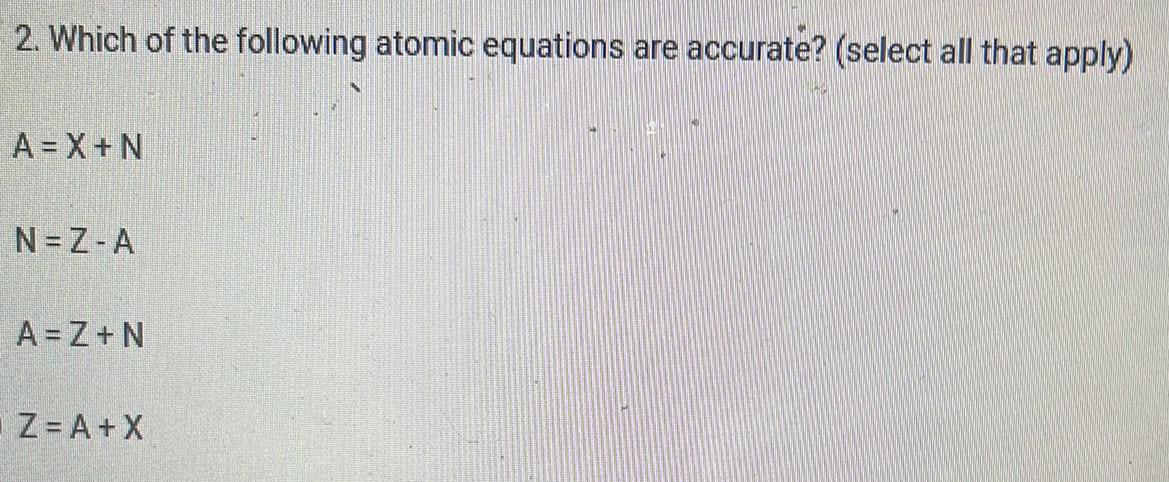Question:

# Which of the following atomic equations are accurate?

Last updated: 8/7/2022Which of the following atomic equations are accurate? (select all that apply) A=X+N N=Z-A A = Z+N Z = A + X